Next: Exercises Up: Ellipsoidal Potential Theory Previous: Introduction

# Analysis

Consider the contribution to the potential at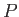from the mass contained within a double cone, whose apex is, and which is terminated in both directions at the body's outer boundary. (See Figure D.1.) If the cone subtends a solid angle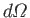then a volume element is written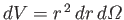, wheremeasures displacement fromalong the axis of the cone. Thus, from standard classical gravitational theory (Fitzpatrick 2012), the contribution to the potential takes the form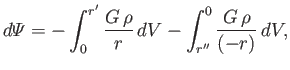(D.2)

where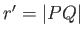,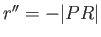, andis the constant mass density of the ellipsoid. Hence, we obtain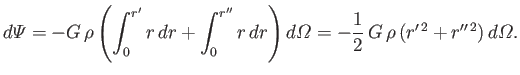(D.3)

The net potential atis obtained by integrating over all solid angle, and dividing the result by two to adjust for double counting. This yields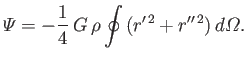(D.4)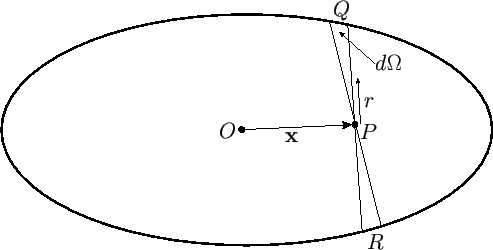From Figure D.1, the position vector of point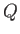, relative to the origin,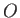, is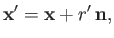(D.5)

where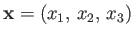is the position vector of point, and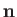a unit vector pointing fromto. Likewise, the position vector of point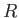is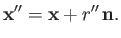(D.6)

However,andboth lie on the body's outer boundary. It follows, from Equation (D.1), that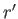and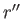are the two roots of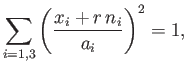(D.7)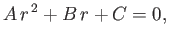(D.8)

where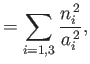(D.9)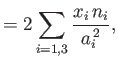(D.10)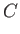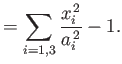(D.11)

According to standard polynomial equation theory (Riley 1974),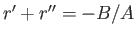, and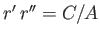. Thus,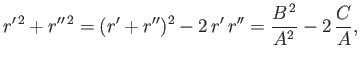(D.12)

and Equation (D.4) becomes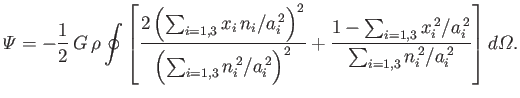(D.13)

The previous expression can also be written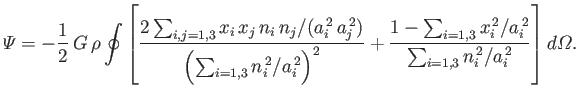(D.14)

However, the cross terms (i.e.,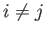) integrate to zero by symmetry, and we are left with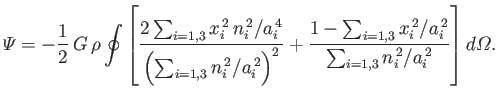(D.15)

Let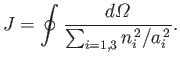(D.16)

It follows that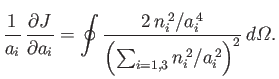(D.17)

Thus, Equation (D.15) can be written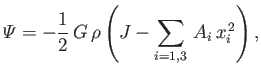(D.18)

where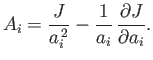(D.19)

At this stage, it is convenient to adopt the spherical angular coordinates,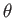and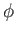(see Section C.4), in terms of which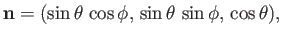(D.20)

and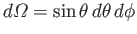. We find, from Equation (D.16), that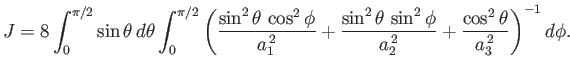(D.21)

Let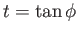. It follows that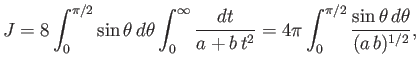(D.22)

where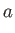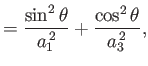(D.23)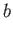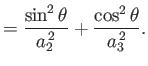(D.24)

Hence, we obtain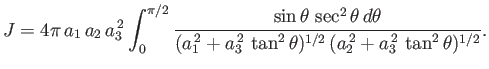(D.25)

Let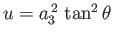. It follows that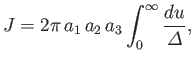(D.26)

where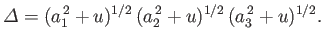(D.27)

Now, from Equations (D.19), (D.26), and (D.27),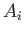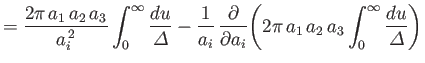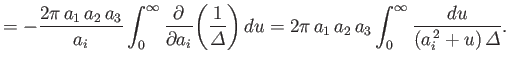(D.28)

Thus, Equations (D.18), (D.26), and (D.28) yield(D.29)

where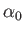(D.30)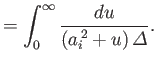(D.31)

Here,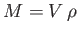and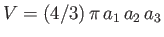are the body's mass and volume, respectively.

The total gravitational potential energy of the body is written (Fitzpatrick 2012)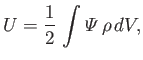(D.32)

where the integral is taken over all interior points. It follows from Equation (D.29) that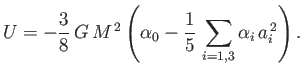(D.33)

In writing the previous expression, use has been made of the easily demonstrated result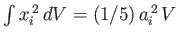. Now,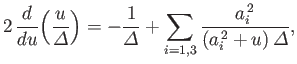(D.34)

so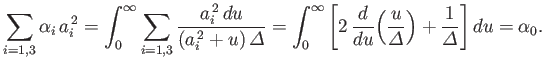(D.35)

Hence, we obtain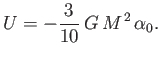(D.36)Next: Exercises Up: Ellipsoidal Potential Theory Previous: Introduction
Richard Fitzpatrick 2016-03-31